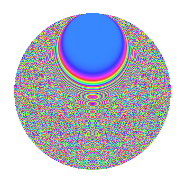# Properties

 Label 1849.2.gLevel 1849 Weight 2 Character orbit g Rep. character $$\chi_{1849}(210,\cdot)$$ Character field $$\Q(\zeta_{21})$$ Dimension 1560 Sturm bound 315

# Related objects

## Defining parameters

 Level: $$N$$ $$=$$ $$1849 = 43^{2}$$ Weight: $$k$$ $$=$$ $$2$$ Character orbit: $$[\chi]$$ $$=$$ 1849.g (of order $$21$$ and degree $$12$$) Character conductor: $$\operatorname{cond}(\chi)$$ $$=$$ $$43$$ Character field: $$\Q(\zeta_{21})$$ Sturm bound: $$315$$

## Dimensions

The following table gives the dimensions of various subspaces of $$M_{2}(1849, [\chi])$$.

Total New Old
Modular forms 2160 2040 120
Cusp forms 1632 1560 72
Eisenstein series 528 480 48

## Trace form

 $$1560q + 10q^{2} + 16q^{3} - 206q^{4} + 17q^{5} + 4q^{6} - 6q^{7} - 18q^{8} + 92q^{9} + O(q^{10})$$ $$1560q + 10q^{2} + 16q^{3} - 206q^{4} + 17q^{5} + 4q^{6} - 6q^{7} - 18q^{8} + 92q^{9} + 7q^{10} + 2q^{11} - 2q^{12} + q^{13} - 18q^{14} + 3q^{15} - 130q^{16} + 11q^{17} - 11q^{18} - 10q^{19} + 3q^{20} + 21q^{21} + 3q^{22} - 3q^{23} - 31q^{24} + 51q^{25} + 15q^{26} + 4q^{27} - 20q^{28} - 9q^{29} - 88q^{30} - 39q^{31} - 48q^{32} + 11q^{33} + 42q^{34} - 11q^{35} - 121q^{36} + 19q^{37} + 21q^{38} + q^{39} + 97q^{40} + 26q^{41} - 2q^{42} - 470q^{44} + 46q^{45} + 61q^{46} + 22q^{47} + 97q^{48} - 48q^{49} + 3q^{50} - 57q^{51} + 12q^{52} + 25q^{53} - 34q^{54} - 14q^{55} - 25q^{56} - 52q^{57} - 64q^{58} - 7q^{59} - 97q^{60} + 14q^{61} - 33q^{62} - 47q^{63} - 20q^{64} - 38q^{65} - 65q^{66} - 65q^{67} - 62q^{68} + 7q^{69} - 47q^{70} + 33q^{71} - 26q^{72} - 29q^{73} + 54q^{74} + 55q^{75} + 39q^{76} + 27q^{77} + 126q^{78} - 7q^{79} - 8q^{80} - 73q^{81} + 54q^{82} + 24q^{83} + 127q^{84} + 56q^{85} - 298q^{87} + 17q^{88} + 19q^{89} + 275q^{90} + 13q^{91} - 6q^{92} + 30q^{93} - 44q^{94} + 9q^{95} + 50q^{96} + 21q^{97} + 5q^{98} + 36q^{99} + O(q^{100})$$

## Decomposition of $$S_{2}^{\mathrm{new}}(1849, [\chi])$$ into newform subspaces

The newforms in this space have not yet been added to the LMFDB.

## Decomposition of $$S_{2}^{\mathrm{old}}(1849, [\chi])$$ into lower level spaces

$$S_{2}^{\mathrm{old}}(1849, [\chi]) \cong$$ $$S_{2}^{\mathrm{new}}(43, [\chi])$$$$^{\oplus 2}$$

## Hecke characteristic polynomials

There are no characteristic polynomials of Hecke operators in the database